# Angles In A Pentagon

A pentagon has 5 sides and is made up of three triangles. Hence, the number of angles in a pentagon are five (in any polygon the number of sides is equal to the number of angles). Below are the different types of polygons namely regular, irregular, and concave pentagon, respectively. Regular pentagon is also called convex pentagon.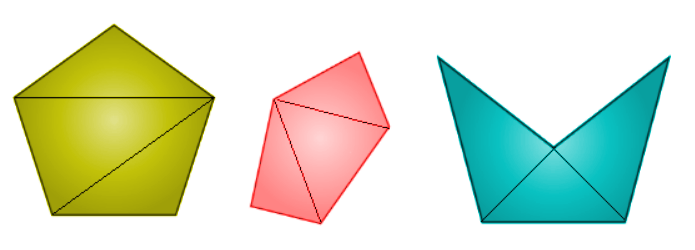## Sum of angles in a pentagon

Pentagon is formed from three triangles, so the sum of angles in a pentagon = 3 × 180°

Sum of the interior angles of a polygon of n sides = (n – 2) × 180° = 540°. This makes a condition for the angles of a pentagon as “angle sum property of pentagon”, this helps in solving many problems related to the angles of pentagon.

## Interior angles in a pentagon

The angles inside of a pentagon which are formed by two adjacent pairs of sides are called interior angles of a pentagon.

Number of sides = Number of vertices = Number of interior angles = 5

Two interior angles that share a common side are called adjacent angles or adjacent interior angles.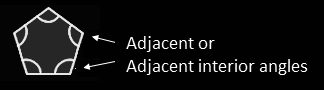Each interior angle will have the respective exterior angle. The sum of interior and exterior angle is equal to the straight angle, i.e. 180°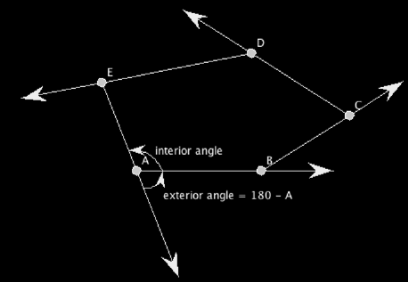### Angles in a regular pentagon

A regular pentagon has all its five sides equal and all five angles are also equal. Hence, the measure of each angle of a regular pentagon is given by the below formula.

Measure of each angle = [(n – 2) × 180°]/n = 540°/5 = 108°

Here, n = Number of sides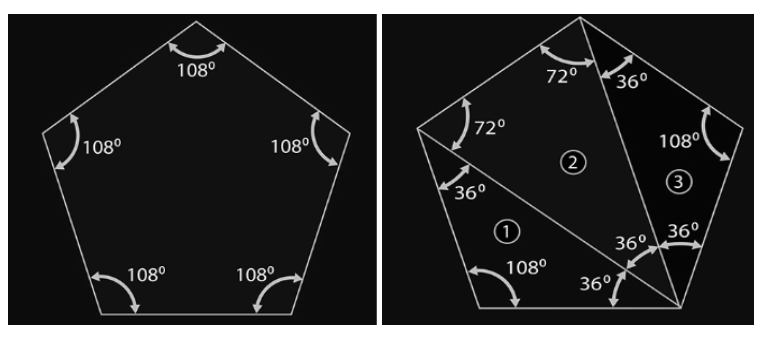Measure of a central angle

The measure of central angle a regular pentagon makes a circle, i.e. total measure is 360°. If we divide pentagon into five congruent triangles, then the angle at one vertex of them will be 72° (360°/5 = 72°).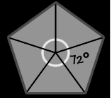### What angles are in a pentagon?

The angles of a pentagon include acute, right and obtuse angles. A pentagon can have at most three right angles. If there are three right angles, then the other two angles will be obtuse angles. All the five angles can be obtuse but all angles cannot be right angles or obtuse angles (since the angle sum property should hold true).

Suppose if all the five angles are right angles, then the sum of the angles = 5 × 90° = 450°

If all the five angles are acute angles, then the sum will be less than 450°.

Hence, this is not true for a pentagon, where the sum of all the angles = 540°

Example 1:

Three angles of a pentagon are 80°, 70° and 100°, then the other two angles can be 145° and 145° or 120° and 180°?

Solution:

Given three angles are 80°, 70° and 100°.

Sum of three angles = 80° + 70° + 100° = 250°

We know that, sum of all the five angles of a pentagon is 540°.

Sum of the other two angles = 540° – 250° = 290°

Now,

145° + 145° = 290°

120° + 180° = 300°

Hence, the other two angles of a pentagon are 145° and 145°.

Example 2:

Find the value of x from the below given figure of pentagon.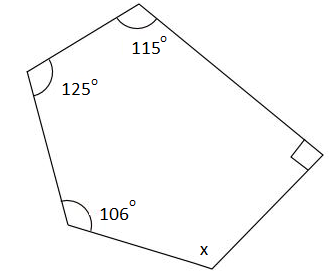Solution:

Given that, one of the angles of a pentagon is a right angle, i.e. 90°.

By angle sum property of a pentagon,

x + 90° + 115° + 125° + 106° = 540°

x + 436° = 540°

x = 540° – 436°

x = 104°

Test your Knowledge on Angles in a Pentagon